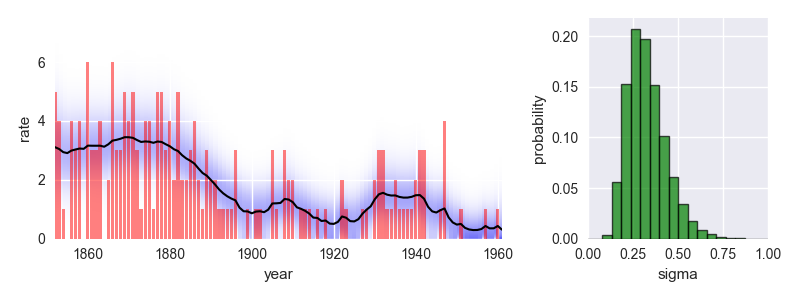Methods

# bayesloopTime series analysis today is an important cornerstone of quantitative science in many disciplines, including natural and life sciences as well as economics and social sciences. Regarding diverse phenomena like tumor cell migration, brain activity and stock trading, a similarity of these complex systems becomes apparent: the observable data we measure – cell migration paths, neuron spike rates and stock prices – are the result of a multitude of underlying processes that act over a broad range of spatial and temporal scales. It is thus to expect that the statistical properties of these systems are not constant, but themselves show stochastic or deterministic dynamics of their own. Time series models used to understand the dynamics of complex systems therefore have to account for temporal changes of the models’ parameters.

bayesloop is a python module that focuses on fitting time series models with time-varying parameters and model selection based on Bayesian inference. Instead of relying on MCMC methods, bayesloop uses a grid-based approach to evaluate probability distributions, allowing for an efficient approximation of the marginal likelihood (evidence). The marginal likelihood represents a powerful tool to objectively compare different models and/or optimize the hyper-parameters of hierarchical models. To avoid the curse of dimensionality when analyzing time series models with time-varying parameters, bayesloop employs a sequential inference algorithm that is based on the forward-backward-algorithm used in Hidden Markov models. Here, the relevant parameter spaces are kept low-dimensional by processing time series data step by step. The module covers a large class of time series models and is easily extensible.

The underlying algorithm of bayesloop has been successfully employed in cancer research, studying the migration paths of invasive tumor cells, see this article.

## Features

• infer time-varying parameters from time series data
• compare hypotheses about parameter dynamics (model evidence)
• create custom models based on SymPy and SciPy
• straight-forward handling of missing data points
• predict future parameter values
• detect change-points and structural breaks in time series data
• employ model selection to online data streams

## Getting started

For a comprehensive introduction and overview of the main features that bayesloop provides, see the documentation.

The following code provides a minimal example of an analysis carried out using bayesloop. The data here consists of the number of coal mining disasters in the UK per year from 1851 to 1962 (see this article for further information).

``````import bayesloop as bl
import matplotlib.pyplot as plt
from matplotlib import gridspec
import seaborn as sns

S = bl.HyperStudy()  # start new data study

# observed number of disasters is modeled by Poisson distribution
L = bl.observationModels.Poisson('rate')
S.setObservationModel(L)

# disaster rate itself may change gradually over time
T = bl.transitionModels.GaussianRandomWalk('sigma',
bl.cint(0, 1.0, 20), target='rate')
S.setTransitionModel(T)

S.fit()  # inference

# plot data together with inferred parameter evolution
plt.figure(figsize=(8, 3))
gs = gridspec.GridSpec(1, 2, width_ratios=[3, 1])

plt.subplot(gs)
plt.xlim([1852, 1961])
plt.bar(S.rawTimestamps, S.rawData,
align='center', facecolor='r', alpha=.5)
S.plotParameterEvolution('rate')
plt.xlabel('year')

# plot hyper-parameter distribution
plt.subplot(gs)
plt.xlim([0, 1])
S.getHyperParameterDistribution('sigma', plot=True,
facecolor='g', alpha=0.7)
plt.tight_layout()
plt.show()
``````This analysis indicates a significant improvement of safety conditions between 1880 and 1900. Check out the documentation for further insights!

## Installation

The easiest way to install the latest release version of bayesloop is via `pip`:

``````pip install bayesloop
``````

Alternatively, a zipped version can be downloaded here. The module is installed by calling `python setup.py install`.

### Development version

The latest development version of bayesloop can be installed from the master branch using pip (requires git):

``````pip install git+https://github.com/christophmark/bayesloop
``````

Alternatively, use this zipped version or clone the repository.

## Dependencies

bayesloop is tested on Python 2.7, 3.5 and 3.6. It depends on NumPy, SciPy, SymPy, matplotlib, tqdm and dill. All except the last two are already included in the Anaconda distribution of Python. Windows users may also take advantage of pre-compiled binaries for all dependencies, which can be found at Christoph Gohlke’s page.

## Optional dependencies

bayesloop supports multiprocessing for computationally expensive analyses, based on the pathos module. The latest version can be obtained directly from GitHub using pip (requires git):

``````pip install git+https://github.com/uqfoundation/pathos
``````

Note: Windows users need to install a C compiler before installing pathos. One possible solution for 64bit systems is to install Microsoft Visual C++ 2008 SP1 Redistributable Package (x64) and Microsoft Visual C++ Compiler for Python 2.7.